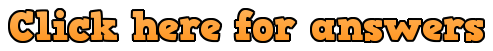Workout

Question 1:

(a)  x = -2  or   x = -4                  (b)   x = -6  or  x = -4             (c)  x = -4    or   x = -10

(d)  x = -5   or   x = 9                  (e)   x = 5   or  x = 7              (f)  x = -1   or   x = 3

(g)  x = -17  or  x = 3                  (h)   x = -2  or  x = 8              (i)  x = 1

Question 2:

(a)  x = -4   or   x = -1                (b)  x = -3   or   x = 6             (c)  x = -4   or   x = 3

(d)  x = 3   or   x = 4                  (e)  x = 3   or   x = 8              (f)   x = -3   or  x = 10

Question 3:

(a)   x = -2 + √7   or    x = -2 – √7

(b)  x = -3 + √19  or   x = -3 – √19

(c)  x = 1 + √6   or   x = 1 – √6

(d)  x = 5 + 2√6   or   x = 5 – 2√6   (note √24 = 2√6)

(e)  x = -4 + √13   or  x = -4 – √13

(f)  x = 4 + √38    or  x = 4 – √38

(g)  x = -10 + √93   or   x = -10 – √93

(h)  x = 6 + √35   or   x = 6 – √35

(i)  x = 15 + 5√13  or  x = 15 – 5√13   (note √325 = 5√13)

Question 4:

(a)  x = -1/2 + √29/2   or    x = -1/2 – √29/2

(b)  x = 3/2 + √5/2    or    x = 3/2 – √5/2

(c)  x = -11/2 + √141/2   or  x = -11/2 – √141/2

(d)  x = 7/2 + √13/2   or   x = 7/2 – √13/2

(e)  x = 1/2 + √201/2   or  x = 1/2 – √201/2

(f)  x = -13/2 + √165/2   or  x = -13/2 – √165/2

Question 5:

(a)  x = 2 – √13    or   x = 2 + √13

(b)  x = -5 + 2√10   or   x = -5 – 2√10

(c)  x = 1/2 – √29/2   or   x = 1/2 + √29/2

(d)  x = -1 + √6   or   x = -1 – √6

(e)  x = -5 + √37   or  x = -5 – √37

(f)  x = -1 – √14   or   x = -1 + √14

Question 6:

(a)   x = -3 + √11   or   x = -3 – √11

(b)   x = -3  or  x = -0.5

(c)   x = -2 + √14/3  or  x = -2 – √14/3

(d)  x = 3/4 + √65/4   or   x = 3/4 – √65/4

(e)  x = -1/5 + √41/5   or   x = -1/5 – √41/5

(f)  x = 1/10 + √11/10  or  x = 1/10 – √11/10

Apply

Question 1:   (a)  (0, 3)     (b)  (-5+√22, 0)  and  (-5-√22, 0)

Question 2:   width = -10 + 10√11 cm    and   length = 10 + 10√11 cm

Question 3:  When solving using completing the square, Abby will get to the point (x+2)^2 = -11.  She will need to √-11, which has no solutions (real ones anyway!)

Question 4:   5√17

Question 5:  a = 6  and   b = -8